### PROC GLM for Unbalanced ANOVA

Analysis of variance, or ANOVA, typically refers to partitioning the variation in a variable’s values into variation between and within several groups or classes of observations. The GLM procedure can perform simple or complicated ANOVA for balanced or unbalanced data.

This example discusses the analysis of variance for the unbalanceddata shown in Table 42.1. The experimental design is a full factorial, in which each level of one treatment factor occurs at each level of the other treatment factor. Note that there is only one value for the cell with A=‘A2’ and B=‘B2’. Since one cell contains a different number of values from the other cells in the table, this is an unbalanced design.

Table 42.1: Unbalanced Two-Way Data

 A1 A2 B1 12, 14 20, 18 B2 11, 9 17

The following statements read the data into a SAS data set and then invoke PROC GLM to produce the analysis.

title 'Analysis of Unbalanced 2-by-2 Factorial';
data exp;
input A $B$ Y @@;
datalines;
A1 B1 12 A1 B1 14     A1 B2 11 A1 B2 9
A2 B1 20 A2 B1 18     A2 B2 17
;

proc glm data=exp;
class A B;
model Y=A B A*B;
run;


Both treatments are listed in the CLASS statement because they are classification variables. A*B denotes the interaction of the A effect and the B effect. The results are shown in Figure 42.1 and Figure 42.2.

Figure 42.1: Class Level Information

 Analysis of Unbalanced 2-by-2 Factorial

The GLM Procedure

Class Level Information
Class Levels Values
A 2 A1 A2
B 2 B1 B2

 Number of Observations Read 7 7

Figure 42.1 displays information about the classes as well as the number of observations in the data set. Figure 42.2 shows the ANOVA table, simple statistics, and tests of effects.

Figure 42.2: ANOVA Table and Tests of Effects

 Analysis of Unbalanced 2-by-2 Factorial

The GLM Procedure

Dependent Variable: Y

Source DF Sum of Squares Mean Square F Value Pr > F
Model 3 91.71428571 30.57142857 15.29 0.0253
Error 3 6.00000000 2.00000000
Corrected Total 6 97.71428571

R-Square Coeff Var Root MSE Y Mean
0.938596 9.801480 1.414214 14.42857

Source DF Type I SS Mean Square F Value Pr > F
A 1 80.04761905 80.04761905 40.02 0.0080
B 1 11.26666667 11.26666667 5.63 0.0982
A*B 1 0.40000000 0.40000000 0.20 0.6850

Source DF Type III SS Mean Square F Value Pr > F
A 1 67.60000000 67.60000000 33.80 0.0101
B 1 10.00000000 10.00000000 5.00 0.1114
A*B 1 0.40000000 0.40000000 0.20 0.6850

The degrees of freedom can be used to check your data. The Model degrees of freedom for afactorial design with interaction are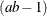, where a is the number of levels of A and b is the number of levels of B; in this case,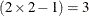. The Corrected Total degrees of freedom are always one less than the number of observations used in the analysis; in this case, 7 – 1 = 6.

The overall F test is significant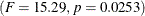, indicating strong evidence that the means for the four different AB cells are different. You can further analyze this difference by examining the individual tests for each effect.

Four types of estimable functions of parameters are available for testing hypotheses in PROC GLM. For data with no missing cells, the Type III and Type IV estimable functions are the same and test the same hypotheses that would be tested if the data were balanced. Type I and Type III sums of squares are typically not equal when the data are unbalanced; Type III sums of squares are preferred in testing effects in unbalanced cases because they test a function of the underlying parameters that is independent of the number of observations per treatment combination.

According to a significance level of 5%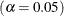, the A*B interaction is not significant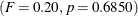. This indicates that the effect of A does not depend on the level of B and vice versa. Therefore, the tests for the individual effects are valid, showing a significant A effect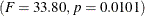but no significant B effect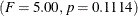.

If ODS Graphics is enabled, GLM also displays by default an interaction plot for this analysis. The following statements, which are the same as in the previous analysis but with ODS Graphics enabled, additionally produce Figure 42.3.

ods graphics on;
proc glm data=exp;
class A B;
model Y=A B A*B;
run;
ods graphics off;


Figure 42.3: Plot of Y by A and B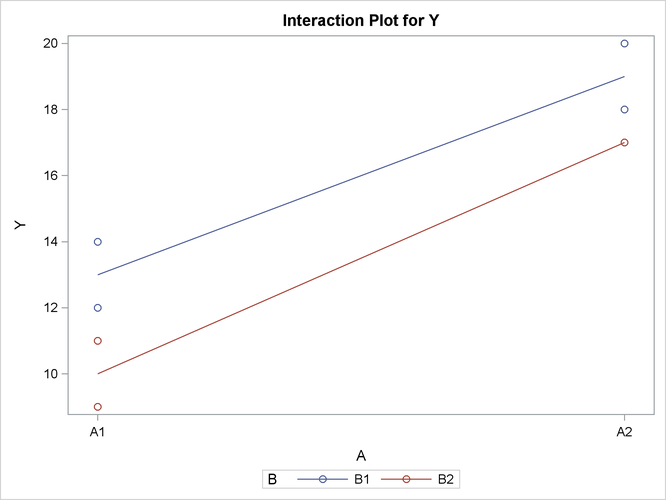The insignificance of the A*B interaction is reflected in the fact that two lines in Figure 42.3 are nearly parallel. For more information about the graphics that GLM can produce, see the section ODS Graphics.